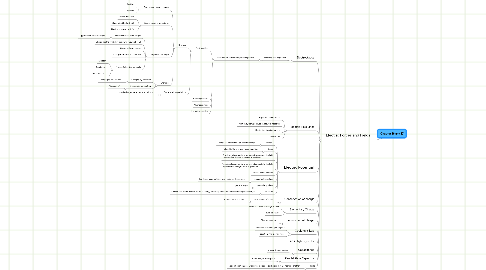# Chapter 16 and 17

Jetzt loslegen. Gratis!Chapter 16 and 17 von## 1. Electric Forces and Fields

### 1.1. Electrostatics

1.1.1. Electricity and Magnetism

1.1.1.1. Introduction to Electricity and Magnetism

1.1.1.1.1. Electrostatics

1.1.1.1.2. Electrodynamics

1.1.1.1.3. Magnetostatics

1.1.1.1.4. Magnetodynamics

### 1.2. Electric Field Lines

1.2.1. Magnitude and Direction

1.2.2. Point away from positives and toward negatives

1.2.3. 5 basic field line distributions

1.2.4. Simulations

### 1.3. Electric Potential

1.3.1. “Voltage”

1.3.1.1. Change in Potential Energy per unit charge

1.3.2. Scalar

1.3.2.1. Related to the energy of a configuration

1.3.3. Positives gain potential when moved opposite to the field Positives naturally go “downhill” in potential

1.3.4. Negatives lose potential when moved opposite to the field Negatives naturally go “uphill” in potential

1.3.5. Va de Graaff Generator

1.3.6. equipotential surface

1.3.6.1. has the same potential at every point on the surface

1.3.8. capacitor

1.3.8.1. a device that stores electric potential energy by storing seperated positive and negative charges

### 1.4. Conservation of charge

1.4.1. total amount of charge

1.4.1.1. universe never changes

### 1.5. Elementary Charge

1.5.1. proton and electron charge is same

1.5.2. build up charge

### 1.6. Polarization of charge

1.6.1. Charges seperate

### 1.7. Coulomb's Law

1.7.1. interaction with charged objects

1.7.2. equation- find electric force

### 1.9. Capacitance

1.9.1. Energy Storage device

### 1.10. Parallel Plate Capacitor

1.10.1. Permittivity of Free Space

### 1.11. dipole

1.11.1. a pair of point charges with equal snd opposite charges that are near one another